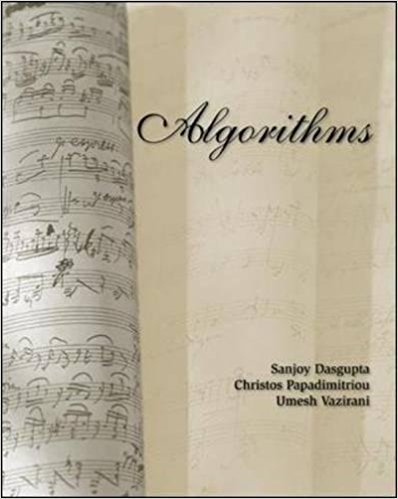×
Log in to StudySoup
Get Full Access to Statistics - Textbook Survival Guide
Join StudySoup for FREE
Get Full Access to Statistics - Textbook Survival Guide

Already have an account? Login here
×
Reset your password

Textbooks / Statistics / Algorithms 1

# Algorithms 1st Edition - Solutions by Chapter## Full solutions for Algorithms | 1st Edition

ISBN: 9780073523408Algorithms | 1st Edition - Solutions by Chapter

Solutions by Chapter
4 5 0 431 Reviews
##### ISBN: 9780073523408

Since problems from 11 chapters in Algorithms have been answered, more than 28625 students have viewed full step-by-step answer. This expansive textbook survival guide covers the following chapters: 11. The full step-by-step solution to problem in Algorithms were answered by , our top Statistics solution expert on 03/08/18, 07:35PM. Algorithms was written by and is associated to the ISBN: 9780073523408. This textbook survival guide was created for the textbook: Algorithms , edition: 1.

Key Statistics Terms and definitions covered in this textbook
• Analysis of variance (ANOVA)

A method of decomposing the total variability in a set of observations, as measured by the sum of the squares of these observations from their average, into component sums of squares that are associated with speciic deined sources of variation

• Analytic study

A study in which a sample from a population is used to make inference to a future population. Stability needs to be assumed. See Enumerative study

• Asymptotic relative eficiency (ARE)

Used to compare hypothesis tests. The ARE of one test relative to another is the limiting ratio of the sample sizes necessary to obtain identical error probabilities for the two procedures.

• C chart

An attribute control chart that plots the total number of defects per unit in a subgroup. Similar to a defects-per-unit or U chart.

• Contrast

A linear function of treatment means with coeficients that total zero. A contrast is a summary of treatment means that is of interest in an experiment.

• Covariance

A measure of association between two random variables obtained as the expected value of the product of the two random variables around their means; that is, Cov(X Y, ) [( )( )] =? ? E X Y ? ? X Y .

• Covariance matrix

A square matrix that contains the variances and covariances among a set of random variables, say, X1 , X X 2 k , , … . The main diagonal elements of the matrix are the variances of the random variables and the off-diagonal elements are the covariances between Xi and Xj . Also called the variance-covariance matrix. When the random variables are standardized to have unit variances, the covariance matrix becomes the correlation matrix.

• Defect concentration diagram

A quality tool that graphically shows the location of defects on a part or in a process.

• Defects-per-unit control chart

See U chart

• Deming

W. Edwards Deming (1900–1993) was a leader in the use of statistical quality control.

• Deming’s 14 points.

A management philosophy promoted by W. Edwards Deming that emphasizes the importance of change and quality

• Distribution free method(s)

Any method of inference (hypothesis testing or conidence interval construction) that does not depend on the form of the underlying distribution of the observations. Sometimes called nonparametric method(s).

• Erlang random variable

A continuous random variable that is the sum of a ixed number of independent, exponential random variables.

• Estimate (or point estimate)

The numerical value of a point estimator.

• Expected value

The expected value of a random variable X is its long-term average or mean value. In the continuous case, the expected value of X is E X xf x dx ( ) = ?? ( ) ? ? where f ( ) x is the density function of the random variable X.

• Experiment

A series of tests in which changes are made to the system under study

• F distribution.

The distribution of the random variable deined as the ratio of two independent chi-square random variables, each divided by its number of degrees of freedom.

• Factorial experiment

A type of experimental design in which every level of one factor is tested in combination with every level of another factor. In general, in a factorial experiment, all possible combinations of factor levels are tested.

• Fraction defective

In statistical quality control, that portion of a number of units or the output of a process that is defective.

• Geometric random variable

A discrete random variable that is the number of Bernoulli trials until a success occurs.